﻿ ES6 Javascript: Part 1

# ES6 Javascript: Part 1

Hi,

Thought of revisiting the basics and writing about that. ES6 is one of the most basic thing which every developer should be knowing. In this series, we will covering complete ES6 end to end. To get started with, I guess arrays will be a good choice. Below are the features, which we will be discussing.

Array Helper Methods:-

• forEach
• map
• filter
• find
• every
• some
• reduce

Now, the same thing we can write ES6 way via forEach.

Therefore, in forEach method, we pass an anonymous function, which is the internal argument to the forEach call. This function gets called one time for every element in the array and hence prints the output. Now, let’s look at some complex example.

Code is self explanatory. Only thing here, instead of writing function, I used arrow convention to replace function and passed in argument. Also, you can see, with ES6 constructs, writing complex programs can be greatly simplified. This will produce the below output.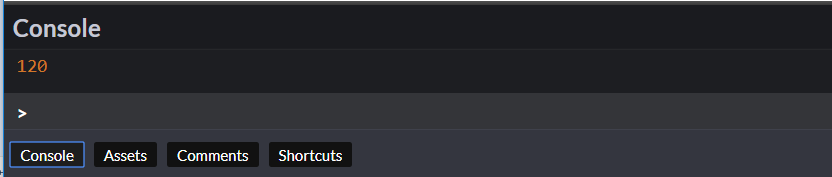Now, let’s go ahead and look at map helper. One of the most common scenarios for using map operator is plucking out values from the object. Let’s consider below scenario, where you have an array of objects with employee set and you need to form a new array with employee last name and designation. In such cases, map becomes very useful.

And, its output will come like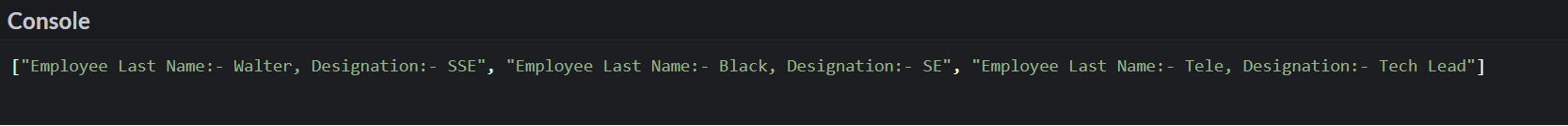Now, let’s go ahead and look at filter helper.

Its output will appear like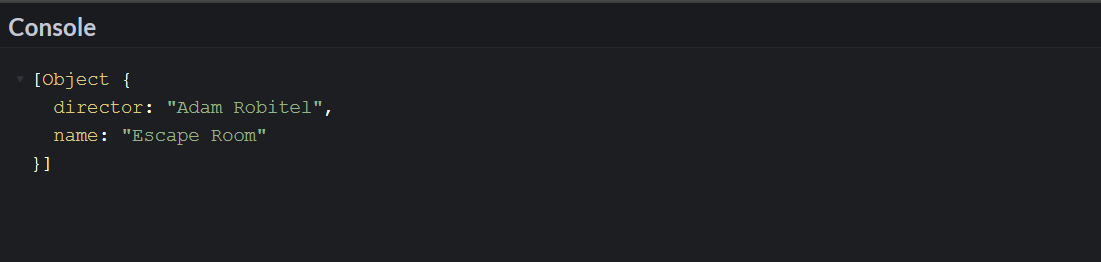Not, let us go ahead and see find in action. I am very sure that most of the guys have used for loop for most of the cases including this one.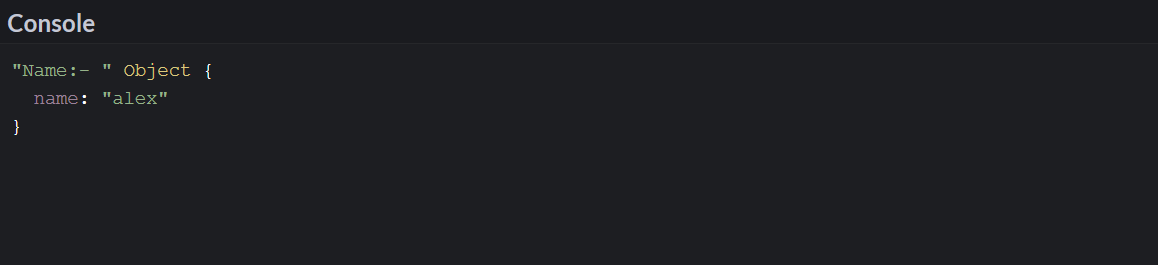But, with find operation, it becomes way easy to scan the code and perform the operation. Now, let’s look at the every and some operator. Here is the example for the same.

This is also straight forward and very useful operator. In the absence of this, you might be writing the same code with for loop with conditions inside.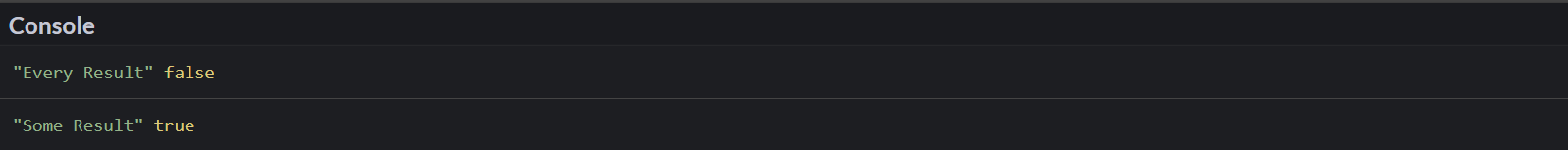Now, let’s go ahead and look at reduce helper.

This example is classic example from reduce use case. Here, I just need to get the sum of numbers. In the first example, I calculated the same using old way and then I applied reducer function. In reducer function, we need to initialize the same as well, hence followed the syntax for the same and then its iterating over the array and then adding the same to sum variable. Finally, I returned the sum in one result variable.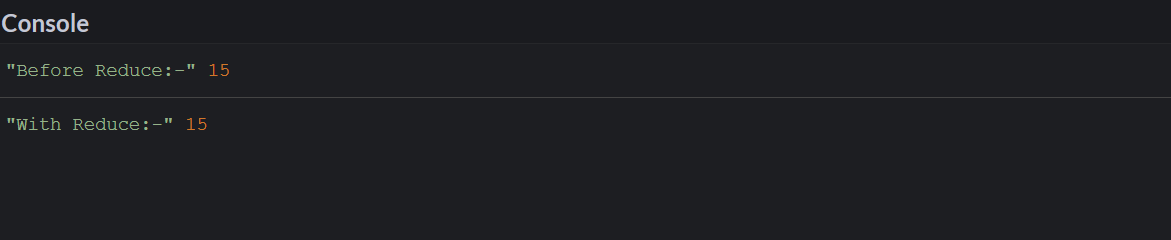In the next section, we will explore more of ES 6 constructs. Till then stay tuned and Happy Coding.

Thanks,
Rahul Sahay,
Happy Coding

Also published on Medium.

Thanks, Rahul Happy Coding